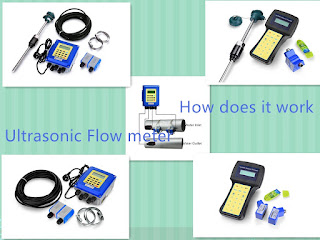### Transit-time Ultrasonic flow meter principle

Transmission (or transit-time) flow meters can be distinguished into in-line (intrusive, wetted) and clamp-on (non-intrusive) varieties.

An ultrasonic flow meter is a type of flow meter that measures the velocity of a fluid with ultrasound to calculate volume flow. Using ultrasonic transducers, the flow meter can measure the average velocity along the path of an emitted beam of ultrasound, by averaging the difference in measured transit time between the pulses of ultrasound propagating into and against the direction of the flow. Ultrasonic flow meters are affected by the acoustic properties of the fluid and can be impacted by temperature, density, viscosity and suspended particulates depending on the exact flow meter. They vary greatly in purchase price but are often inexpensive to use and maintain because they do not use moving parts, unlike mechanical flow meters.
Time transit flow meter

Ultrasonic flow meters measure the difference of the transit time of ultrasonic pulses propagating in and against flow direction. This time difference is a measure for the average velocity of the fluid along the path of the ultrasonic beam. By using the absolute transit times both the averaged fluid velocity and the speed of sound can be calculated.
Using the two transit times $t_{up}$and $t_{down}$and the distance between receiving and transmitting transducers $L$and the inclination angle $\alpha$one can write the equations:and $c={\frac {L}{2}}\;{\frac {t_{up}+t_{down}}{t_{up}\;t_{down}}}$where $v$is the average velocity of the fluid along the sound path and $c$is the speed of sound.Transit-time Ultrasonic flow meter principle